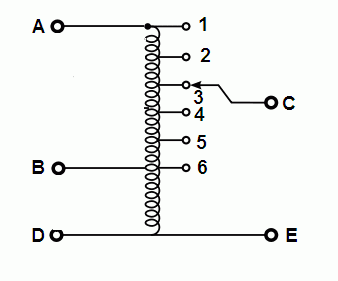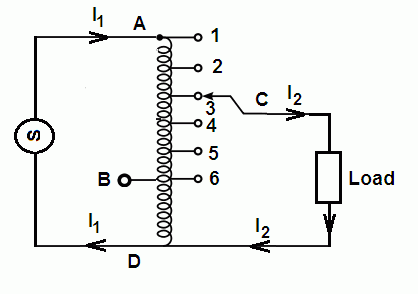# Auto Transformer Working Principle

The auto transformer working principle and construction is similar to that of conventional two winding transformers. However, it differs in the way in which the primary and the secondary are inter-related.

In a two-winding transformer, primary and secondary are only magnetically linked by a common core but are completely insulated from each other. But in the case of an auto transformer windings are connected electrically as well as magnetically.

It consists of only one winding wound on a laminated magnetic core, with a rotary movable contact. The same auto transformer can be used as a step-down or a step-up transformer.# Auto Transformer Working Principle

The circuit diagram of an auto transformer is shown in Figure. When the single phase AC supply is connected between A and D terminals and output is taken from C and E terminals, this auto transformer will operate as a step-down transformer.

Because the number of turns in winding between A and D terminal (i.e. primary winding) is more than the number of turns in winding between C and E terminal (i.e. secondary winding).

On the other hand, when the single phase AC supply is connected between B and D terminals and output is taken from C and E terminals, the same auto transformer will operate as a step-up transformer.

Because the number of turns in winding between B and D terminal (i.e. primary winding) is less than the number of turns in winding between C and E terminal (i.e. secondary winding). We can make small variations in output voltage by taking the output from different tapings of the auto transformer.The current in the winding section of an auto transformer, which is common to both the windings (CD) is minimum (I1I2). Therefore, the cross-sectional area of that winding wire is minimum.

## Electrically Transformed Energy in Autotransformer

In an autotransformer, energy is transformed into the load by two means, electrically as well as magnetically (or inductively). It can be proved that

power transformed inductively = input power(1 − K)

and the power transformed electrically = K × input power

## Saving of Copper in an Autotransformer

It is obvious that the weight of copper required in an autotransformer will be less than that of an ordinary two winding transformer. It can be proved mathematically that the weight  of copper  required in an autotransformer (Wa) will be:

Wa = (1 − K) × Wo

∴ Saving = Wo − Wa
= Wo − (1 − K) Wo = KWo

∴ Saving = K × Wo

Where Wa = weight of Cu in autotransformer,
Wo = weight of Cu is an ordinary transformer,
K = transformation ratio.

It is clear, saving will increase as K approaches unity.

## Advantages | Disadvantages | Applications of Auto transformer

Advantages of an Auto transformer

• Continuously varying voltage can be obtained.
• It needs less copper and is more efficient than a two-winding transformer of same ratings.

Disadvantages of an Auto transformer
If the winding (CE) breaks (open circuited) then the transformer action is lost and full primary voltage appears across the output. It can be harmful to the load when we are using an auto transformer as a step-down transformer. That is why an auto transformer is used for only making small variations in output voltage while using as a step-down transformer.

One another major disadvantage of an autotransformer is that the secondary is not isolated electrically from its primary. When we are using it as a step-down transformer, the secondary may cause severe electrical shock, even if it making a very small voltage (say 25 V). Because it is electrically not isolated from mains (i.e. connected to the mains).

To understand these concepts more clearly, suppose we want to get a 30 AC supply form the 220 V mains. We can get 30 V AC supply by using a 220/30 V step down transformer or by a 220/30 V autotransformer.
But the latter option is generally avoided because:

• Saving in copper will be very small.
• If any fault occurs, 220 V will appear across the secondary terminals and will destroy the devices connected to the secondary.
• When our system is working properly i.e. giving 30 V supply, even then anybody touches the secondary terminal of the transformer (30 V) can get a severe electric shock is some situations because he is not isolated from the mains.

Whereas when we use a step-down transformer, we can easily touch the secondary terminal of the operating transformer because its voltage level is very low (30 V) and its primary and secondary is completely isolated electrically from each other. That is, there is no electrical connection between the primary and the secondary. The power is transferred from one circuit to the second circuit only by magnetic flux.
Applications of an Auto transformer
The auto transformers are used

• as starters for induction motors and synchronous motors which are known as auto transformer starters.
• in labs for obtaining a continuously varying voltage.
• in voltage stabilizers as regulating transformers.
• as booster transformer to raise the voltage in AC feeders.

Thanks for reading about auto transformer working principle.

## Transformer | All Posts

© https://yourelectricalguide.com/ working principle of autotransformer pdf.